Next: 3. Reference Manual Up: 2. User's Guide Previous: 15. The Iota Constructor

Subsections

# 16. Syntax: Parsing and Printing

The purpose of this chapter is threefold:

1.
To explain the relation between syntax and IMPS expressions.
2.
To explain the default syntax, which we refer to as the string syntax,'' and briefly to explain how you can extend or modify this syntax.
3.
To explain how you can redefine the syntax altogether.16.1

# 16.1 Expressions

As explained in Chapter 7, expressions are variables, constants, and compound expressions formed by applying constructors to other expressions. Expressions can thus be represented as Lisp s-expressions:

• Formal symbols (i.e., constants or variables) correspond to atoms.
• Compound expressions correspond to certain lists of the form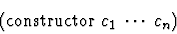where each ci is itself an expression.
In the implementation, an expression is a data structure which also caches a large amount of information such as the free and bound variables of the expression, the constants contained in the expression, the home language of the expression, and so on. For the IMPS user, an expression is typically something which can be represented as text, for instance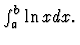The correspondence of an expression as a data structure to an external representation for input or output is determined by the user syntax which is employed. IMPS allows multiple user syntaxes, so for example, the syntax that is used for reading in expressions (usually text) may be different from the syntax used to display expressions (which could be text or text interspersed with TEX commands which would make the output suitable for input to the TEX processor.) This flexible arrangement means users can freely change from one syntax to another, even during the course of an interactive proof.

# 16.2 Controlling Syntax

The process of building an IMPS expression from user input can be broken up into two steps:

1.
Parsing a string into an s-expression s.
2.
Building an IMPS expression from the s-expression s.
Similarly, the process of displaying an expression also consists of two parts:
1.
Building an s-expression s from an IMPS expression.
2.
Providing some representation of s as a string.
The phases string to s-expression'' for reading and s-expression to string'' for printing are under direct user control. They can be modified by resetting the following switches:
• (imps-reader) is a T procedure which takes a language and a port and returns an s-expression. If proc is a procedure of this type, you can reset the imps-reader switch by evaluating
   (set (imps-reader) proc)

• (imps-printer) is a procedure which takes an s-expression and a port and prints something to this port. If proc is a procedure of this type, you can reset the imps-printer switch by evaluating
   (set (imps-printer) proc)

To write your own reader and printer procedures, you will have to do some programming in the T language. However, some reader and printer procedures are particularly easy to write. For example to write your own reader and printer for s-expression syntax, you could evaluate the following s-expressions:
   (set (imps-reader)

   (set (imps-printer)
(lambda (s-exp port) (pretty-print s-exp port)))


In the next section we describe the default syntax which is similar to the syntax of some computer algebra systems.

# 16.3 String Syntax

We begin with some notation: if Term'' is a class of strings,

 Term* = zero or more comma-separated members of Term Term+ = one or more comma-separated members of Term.

A string is accepted by the string syntax exactly when IMPS can build an s-expression s from it. Otherwise, IMPS will give you a parsing error. However, to say the string is accepted does not mean that the system can build an IMPS expression from s. A number of things could go wrong:

• An atom in s has no sort specification, so that IMPS assumes it is the name of a constant, but no such constant exists in the language.
• The arguments of a function are of the wrong type, or there are too many or too few arguments.
• A constructor was given components of the wrong type, or the number of components is wrong.

The tables 16.1, 16.2, and 16.3 are a description of those sequences of characters accepted by the string syntax. This description is fairly close to a BNF grammar, but fails to be one since some of the expression categories (such as the category of binary infix operators or the category of quasi-constructors) are only specified partially or not at all. This is because these expression categories depend on parts of the state of the IMPS system, which are likely to change from user to user.

We also want to stress that these tables say nothing about how strings are parsed into nested lists (and much less about what the strings mean mathematically.) For example, one cannot say how argument associations are disambiguated from these tables alone. The table of operator precedences in the next section specifies this information. The syntax tables are thus of limited value and should only be consulted to get a very rough idea of what expressions look like.

Table 16.1: Description of String Syntax 1
 Exp ::= Aexp Oxp(Oxp*) Function application Exp BInfxOp Exp Binary infix application (Oxp) Parenthesized Oxp Exp oo Exp Composition Oxp =Oxp Oxp ==Oxp not Exp Exp and Exp Exp or Exp Exp implies Exp Exp iff Exp Binder(Binding* , Exp) Binders (including with) iota(Atom:Sort, Exp) if(Exp, Exp, Exp+) Requires an odd number of Exp Qc{Exp+} Prefix quasi-constructor Exp Qc{Exp+,Sort} Prefix quasi-constructor Exp ?Sort Undefined from sort #(Exp,Sort) Sort definedness assertion #(Exp) Definedness assertion Oxp ::= Exp Operator or expression PrefxOp LogfxOp BInfxOp PostfxOp Aexp ::= Num Numerical constants Atom Variables & other constants

Table 16.2: Description of String Syntax 2
 Sort ::= Asort These are base sorts [Sort,Sort+] N-ary function sort constructor. Binder ::= lambda forall forsome with Binding ::= Sortdecl+ Sortdecl ::= Atom+ : Sort PrefxOp ::= comb Combinatorial coefficients lim Limit operator for sequences LogfxOp ::= - BInfxOp ::= * + < <= i-in i-subset PostfxOp ::= !

Table 16.3: Description of Atoms
 Atom ::= TextChar followed by TextChar's or Digit's SpecialSeq SpecialSeq followed by _ and TextChar's or Digit's TextChar ::= a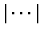z | _ |% | \$ | & Digit ::= 09 SpecialSeq ::= ^ | * | + | - | ^^ | ** | ++ | ! | < | <= | > | >=

# 16.4 Parsing

The process of building an s-expression from an input string, itself breaks up into two parts:

• Tokenization of the input string. This can be thought of as dividing the input string into a list of substrings called tokens.

• Parsing of the list of tokens. This can be thought of as transforming a linear structure of tokens into a tree structure of tokens.

The table below gives some examples of how a string s is translated into an s-expression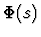according to the string syntax.
 String Reads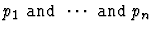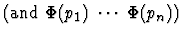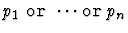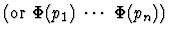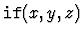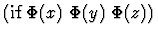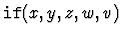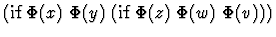56(t,s)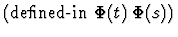57(t)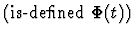x + y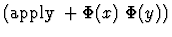x - y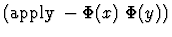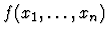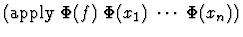To build an s-expression from the input tokens, it is necessary to disambiguate the tokens which are operators16.2 from the tokens which are arguments. The association of tokens to operators is determined by the operator binding powers. For example, the string x+2*3 is parsed as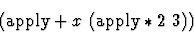because the operator * has a higher binding power than +. Table 16.4 is a list of the bindings of some common operators.

Table 16.4: Operator Binding Powers
 Operator Binding + 100 - 110 * 120 / 121 ^ 140 ! 160 = 80 == 80 i-subset 101 i-in 101 > 80 >= 80 < 80 <= 80 not 70 iff 65 implies 59 and 60 or 50

# 16.5 Modifying the String Syntax

The string syntax can be modified or extended using the def-forms def-parse-syntax and def-print-syntax. The use of def-forms is documented in Chapter 17.

# 16.6 Hints and Cautions

1.
A parsing error is indicated by an error message
   **Error: Parsing error:

This means IMPS was unable to build an s-expression from your input. IMPS will provide some indication as to where the error occurred. Following are some examples of common parsing errors:
   > (qr "with(a,b:rr a=b)")
** Error: Parsing error: Illegal token A encountered.
with(a,b:rr a <<== =b).

This error occurred because the variable sort specifications have to be followed by a comma.
   > (qr "forall(a,b:rr,a=b")
** Error: Parsing error: Expecting ":" or "," or ")".
forall(a,b:rr,a=b <<== .

This error results from a missing right parenthesis.
2.
An error message of one of the following kinds is usually an indication that the current theory (this is the value of the switch (current-theory)) is different from what you expected. This typically happens when you begin an interactive proof of a formula without having set the current theory to its desired value.
   > (qr "with(x:rr,x=1)")
** Error: QUANTIFIER-DECODE-VAR-SUB-LIST:
RR is not a sort in
#{IMPS-basic-language 12: THE-KERNEL-LANGUAGE}.

This error message indicates that IMPS was unable to build an expression in the current theory because there is no sort named rr.
   > (qr "with(x:ind, x+x)")
** Error: SEXP->EXPRESSION-1:
Cannot locate + in
#{IMPS-basic-language 12: THE-KERNEL-LANGUAGE}.

This error message indicates that IMPS was unable to build an expression in the current theory because there is no constant named +. To remedy an error of the above type, reset the (current-theory) switch by using the menu.Next: 3. Reference Manual Up: 2. User's Guide Previous: 15. The Iota Constructor